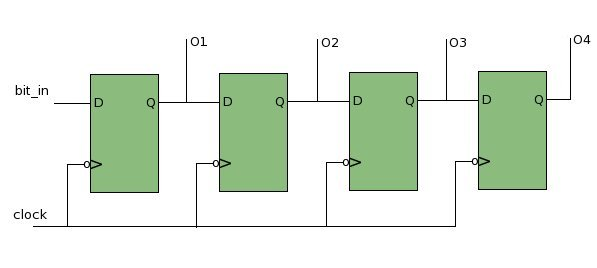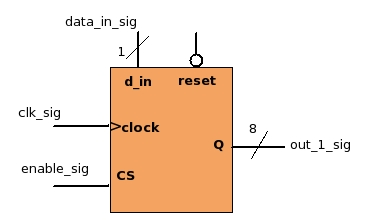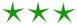black nike shoes roshe cheapnicesports.com

# Coding Shift Register

Coding shift register is quite a simple task. In actual hardware the shift register is implemented as a sequence of flip-flops connected in a series, where the data keeps shifting from one flip-flop to another with every clock edge. As shown in 4-bit shift-register below.Lets see how a 8-bit shift-register implemented in VHDL...

8 bit Shift-RegisterVHDL Code

```1) shift_reg : PROCESS(clk_sig, reset_sig)
2) BEGIN
3)      If(reset_sig = '0') THEN
4)         out_1_sig <= "00000000";
5)      ELSIF(clk_sig 'event AND clk_sig = '1') THEN
6)         IF (enable_sig = '1') THEN
8)           out_1_sig(7 DOWNTO 1) <= out_1_sig(6 DOWNTO 0);
7)           out_1_sig(0) <= data_in_sig;
9)         END If;
10)     END If;
11) END PROCESS;

```

From the above code it is quite clear that first the 7 bits (6 downto 0) are placed at (7 downto 1) and the input bit "data_in_sig" is placed at 0th bit. This is same as shifting each bit by one position.

Here note that the MSB bit (7th) will be lost, every time a new bit enters the shift register.